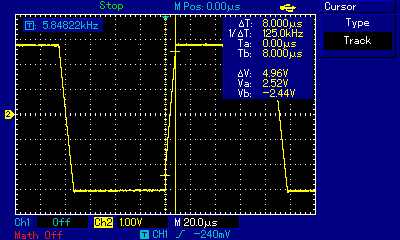# Slew Rate

The slew rate is the maximum change in voltage per unit time. It is usually measured in volts per microsecond (Vμs-1). This is an important metric in certain electronic circuits such as amplifiers.

## Example

A LM741 opamp was put into unity gain mode and connected to a ± 10V power supply, a signal generator, and an oscilloscope. The signal generator was configured to output a square wave. The generator's frequency was set then the output voltage was adjusted until the the slope of the rising edge on the opamp's output pin was at an angle where the slew rate could be easily measured. To calculate the rate, the 10% and 90% points on a rising edge were chosen, and the difference in voltage between these two points was divided by the difference in time between these two points.

According to the LM741 data-sheet, the slew rate should typically be 0.5 Vμs-1. From the capture of the oscilloscope's screen, showing the two cursors positioned at the 10% and 90% points, we see that ΔT is 8μs, and ΔV is 4.96V. This gives us a slew rate of 4.96/8 = 0.62 Vμs-1 which is much better than what the data-sheet promised.## References

LM741 Operational Amplifier.Texas Instruments, 2013.